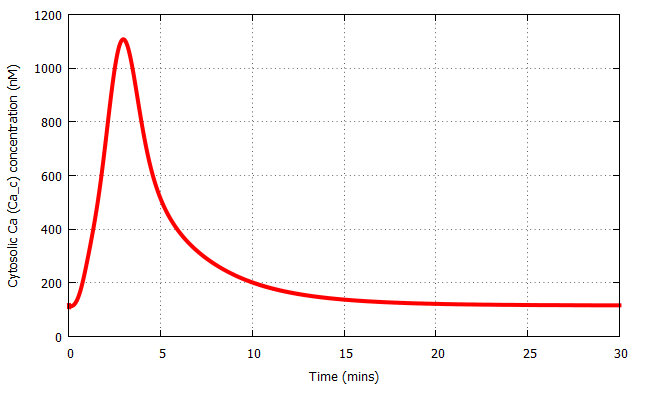## Koo2013 - Shear stress induced calcium influx and eNOS activation - Model 1Model Identifier
BIOMD0000000464
Short description
Format
SBML (L2V4)
Related Publication
• In silico modeling of shear-stress-induced nitric oxide production in endothelial cells through systems biology.• Koo A, Nordsletten D, Umeton R, Yankama B, Ayyadurai S, García-Cardeña G, Dewey CF Jr
• Biophysical journal , 5/ 2013 , Volume 104 , pages: 2295-2306 , PubMed ID: 23708369
• Department of Biological Engineering, Massachusetts Institute of Technology, Cambridge, MA, USA.
• Nitric oxide (NO) produced by vascular endothelial cells is a potent vasodilator and an antiinflammatory mediator. Regulating production of endothelial-derived NO is a complex undertaking, involving multiple signaling and genetic pathways that are activated by diverse humoral and biomechanical stimuli. To gain a thorough understanding of the rich diversity of responses observed experimentally, it is necessary to account for an ensemble of these pathways acting simultaneously. In this article, we have assembled four quantitative molecular pathways previously proposed for shear-stress-induced NO production. In these pathways, endothelial NO synthase is activated 1), via calcium release, 2), via phosphorylation reactions, and 3), via enhanced protein expression. To these activation pathways, we have added a fourth, a pathway describing actual NO production from endothelial NO synthase and its various protein partners. These pathways were combined and simulated using CytoSolve, a computational environment for combining independent pathway calculations. The integrated model is able to describe the experimentally observed change in NO production with time after the application of fluid shear stress. This model can also be used to predict the specific effects on the system after interventional pharmacological or genetic changes. Importantly, this model reflects the up-to-date understanding of the NO system, providing a platform upon which information can be aggregated in an additive way.
Contributors
Andrew Koo

is
BioModels Database MODEL1302180003
BioModels Database BIOMD0000000464
isDescribedBy
PubMed 23708369
hasTaxon
Taxonomy Homo sapiens
hasPart
Gene Ontology calcium ion import
Reactome eNOS synthesizes NO

Curation status
Curated

Original model(s)
Wiesner, T. F., B. C. Berk, and R. M. Nerem. 1996. A mathematical model of cytosolic calcium dynamics in human umbilical vein endothelial cells. Am J Physiol 270:C1556-1569.

Tags
Name Description Size Actions

### Model files

BIOMD0000000464_url.xml SBML L2V4 representation of Koo2013 - Shear stress induced calcium influx and eNOS activation - Model 1 68.17 KB Preview | Download

• Model originally submitted by : Andrew Koo
• Submitted: Feb 18, 2013 8:19:30 PM
##### Revisions
Legends
: Variable used inside SBML models

Species
Species Initial Concentration/Amount
s7 0.0 1E-9*mol
Ca s

calcium(2+)
2830000.0 1E-9*mol
Ca c

calcium(2+) ; cell
117.2 1E-9*mol
Ca B

cell ; calcium(2+)
3870.0 1E-9*mol
s5 0.0 1E-9*mol
IP3

1D-myo-inositol 1,4,5-trisphosphate(6-)
0.0 1E-9*mol
s8 0.0 1E-9*mol
s9 0.0 1E-9*mol
Reactions
Reactions Rate Parameters
(s7) => (IP3)

([s7]) => ([1D-myo-inositol 1,4,5-trisphosphate(6-)])
k1*(R_T-half*R_T*(exp((-s12)/tau_I)+exp((-s12)/tau_II)+(exp((-s12)/tau_I)-exp((-s12)/tau_II))*(tau_I+tau_II)/(tau_I-tau_II)))*s3/(K1+s3)

k1*(R_T-half*R_T*(exp((-[TimeT])/tau_I)+exp((-[TimeT])/tau_II)+(exp((-[TimeT])/tau_I)-exp((-[TimeT])/tau_II))*(tau_I+tau_II)/(tau_I-tau_II)))*[calcium(2+); cell]/(K1+[calcium(2+); cell])
tau_I = 66.0 substance; K1 = 0.0 substance; tau_II = 0.01 substance; k1 = 6.0E-4 sub_sec; half = 0.5 dimensionless; R_T = 44000.0 dimensionless
(s5) => (Ca_s)

([s5]) => ([calcium(2+)])
k_CCE*(fracK*Cao/(K3+Cao)-s2)*(s1-s2)

k_CCE*(fracK*Cao/(K3+Cao)-[calcium(2+)])*([calcium(2+); extracellular region]-[calcium(2+)])
fracK = 7071067.81 substance; Cao = 100.0 substance; K3 = 150.0 substance; k_CCE = 0.0 inv_sec_sub
(Ca_s) => (Ca_c)

([calcium(2+)]) => ([calcium(2+); cell])
(k3*k_CICR*s3/(K_CICR+s3)*(s6/(K2+s6))^3*s2-k4*(s3/(K3+s3))^2)+k5*s2*s2

(k3*k_CICR*[calcium(2+); cell]/(K_CICR+[calcium(2+); cell])*([1D-myo-inositol 1,4,5-trisphosphate(6-)]/(K2+[1D-myo-inositol 1,4,5-trisphosphate(6-)]))^3*[calcium(2+)]-k4*([calcium(2+); cell]/(K3+[calcium(2+); cell]))^2)+k5*[calcium(2+)]*s2
K_CICR = 0.0 substance; K3 = 150.0 substance; k5 = 5.0E-11 inv_sec_sub; k_CICR = 1.0 dimensionless; k4 = 2500.0 sub_sec; k3 = 3.32 inv_sec; K2 = 200.0 substance
(Ca_B) => (Ca_c)

([cell; calcium(2+)]) => ([calcium(2+); cell])
(-k6)*s3*(B_T-s4)+k7*s4

(-k6)*[calcium(2+); cell]*(B_T-[cell; calcium(2+)])+k7*[cell; calcium(2+)]
B_T = 120000.0 substance; k6 = 0.05 inv_sec_sub; k7 = 150.0 inv_sec
(Ca_c) => (s9)

([calcium(2+); cell]) => ([s9])
dot_Vhi*s3^4/(K_hi^4+s3^4)

dot_Vhi*[calcium(2+); cell]^4/(K_hi^4+[calcium(2+); cell]^4)
dot_Vhi = 2380.0 sub_sec; K_hi = 380.0 substance
(Ca_c) => (s10)

([calcium(2+); cell]) => ([s10])
dot_Vex*s3/(K5+s3)

dot_Vex*[calcium(2+); cell]/(K5+[calcium(2+); cell])
K5 = 321.0 substance; dot_Vex = 9165.0 sub_sec
(s11) => (Ca_c)

([s11]) => ([calcium(2+); cell])
dot_q_inpass

dot_q_inpass
[]
(IP3) => (s8)

([1D-myo-inositol 1,4,5-trisphosphate(6-)]) => ([s8])
k2*s6

k2*[1D-myo-inositol 1,4,5-trisphosphate(6-)]
k2 = 1.0 inv_secCurator's comment:
(added: 19 Aug 2013, 13:55:30, updated: 19 Aug 2013, 13:55:30)
The paper describes four sub-models and an integrated version of the four sub-models. This model corresponds to Model 1 - Shear stress induced calcium influx and eNOS activation. Figure 3A of the paper is reproduced here using Copasi 4.10 (Build 55).